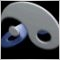# Whats Wrong428

Hi All,

whats wrong with this code. I just want to get long as the last bar closes above the last 20 bars, and RSI is above 14.

extern int Quant_Bars=15;
extern int LongRSI = 50;
extern int ShortRSI = 50;
extern double TakeProfit = 100;
extern double Lots = 1;
extern double TrailingStop = 100;
int start()
{
//----Identify the highest and lowest of the last N bars
int i,ticket; // Bar number
double Minimum=Bid, // Minimal price
Maximum=Bid; // Maximal price

for(i=0;i<=Quant_Bars-1;i++) // From zero (!) to..
{ // ..Quant_Bars-1 (!)
if (Low[i]< Minimum) // If < than known
Minimum=Low[i]; // it will be min
if (High[i]> Maximum) // If > than known
Maximum=High[i]; // it will be max
}
Alert("For the last ",Quant_Bars, // Show message
" bars Min= ",Minimum," Max= ",Maximum);

//Place Orders

if((iRSI(NULL,0,14,PRICE_CLOSE,2) <= LongRSI) && (iRSI(NULL,0,14,PRICE_CLOSE, 1) > LongRSI)&& iClose(NULL,PERIOD_H1,0)>Maximum)
{
if(ticket>0)
{
}
else Print("Error opening BUY order : ",GetLastError());

return(0);
}
//----
return(0);
}
//+------------------------------------------------------------------+428

Sorry, RSI above 50 and last bar close above last 15 bars high.2646

iClose(...,...,0) can't be higher than maximum if you include that close in the calculation of maximum.428

phy.........didn't get your point. m just a newbie here. Can you let me what exactly I need to change in the above code. ?2646

Try

if((iRSI(NULL,0,14,PRICE_CLOSE,2) <= LongRSI) && (iRSI(NULL,0,14,PRICE_CLOSE, 1) > LongRSI)&& iClose(NULL,PERIOD_H1,0)>=Maximum)428

Somehow its not working. I don't know how to workaround this problem.

Another question, about swapping the trade. Say a short trade at RSI<=30 is close, and instantly a long tride is triggered, unless RSI <=70.